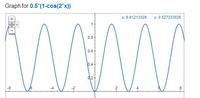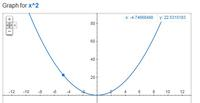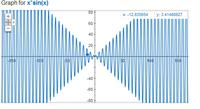# Even function and odd function

Status
Not open for further replies.

#### subbuindia

##### Member level 5
The multiplication of two odd functions is an even function. How? Please, explain with an example?

a(x) = b(x) * c(x)

Now,
b(-x) = -b(x) and c(-x) = -c(x) <--- since b(x) and c(x) are odd functions

So,
a(-x) = b(-x) * c(-x) = -b(x) * -c(x) = b(x) * c(x) = a(x)
so,
a(-x) = a(x) ie even function

•albbg

### albbg

Points: 2
if we have two functions
a(x)=sin(x)
b(x)=sin(x) also
sin(x) is odd function
c(x)=a(x)*b(X)
(multiplying two odd functions
c(x)= (sin(x))^2 (squared sin(x))
we can write it
C(x)=.5*(1- cos(2x))

when u graph function like that you will find it even (attached the graph)

take examples as u want
suggested ramp signal
a(X)=x
and
b(x)=x

c(x)=a(x)*b(X)=x^2 which obviously an even function (attached figure 2) (which is symmetric around the vertical axes then it is even function)

now suggest
a(x)=x
b(x)=sin(x)
c(x)=x*sin(x) see figure 3 (which is symmetric around the vertical axes then it is even function)•subbuindia

Points: 2